# Kinematics 2D - JEE Main Previous Year Questions with Solutions

JEE Main Previous Year Question of Physics with Solutions are available here. Practicing JEE Main Previous Year Papers Questions of Physics will help all the JEE aspirants in realizing the question pattern as well as help in analyzing their weak & strong areas. Get detailed Class 11th &12th Physics Notes to prepare for Boards as well as competitive exams like IIT JEE, NEET etc. eSaral helps the students in clearing and understanding each topic in a better way. eSaral is providing complete chapter-wise notes of Class 11th and 12th both for all subjects. Besides this, eSaral also offers NCERT Solutions, Previous year questions for JEE Main and Advance, Practice questions, Test Series for JEE Main, JEE Advanced and NEET, Important questions of Physics, Chemistry, Math, and Biology and many more. Download eSaral app for free study material and video tutorials. Simulator Previous Years AIEEE/JEE Mains Questions
Q. A particle is moving with velocity $\overrightarrow{\mathrm{v}}=\mathrm{K}(\mathrm{y} \hat{\mathrm{i}}+\mathrm{x} \hat{\mathrm{j}})$ where K is a constant. The general equation for its path is : (1) $\mathrm{y}^{2}=\mathrm{x}^{2}+$ constant (2) $\mathrm{y}=\mathrm{x}^{2}+$ constant (3) $\mathrm{y}^{2}=\mathrm{x}+$ constant (4) xy = constant [AIEEE - 2010]
Ans. (1) $\overrightarrow{\mathrm{v}}=\mathrm{k}(\mathrm{y} \hat{\mathrm{i}}+\mathrm{x} \hat{\mathrm{j}})$ $\overrightarrow{\mathrm{v}}=\mathrm{v}_{\mathrm{x}} \hat{\mathrm{i}}+\mathrm{v}_{\mathrm{y}} \hat{\mathrm{j}}$ $\overrightarrow{\mathrm{v}}=\frac{\mathrm{d} \mathrm{x}}{\mathrm{dt}} \hat{\mathrm{i}}+\frac{\mathrm{dy}}{\mathrm{dt}} \hat{\mathrm{j}}$ $\frac{d x}{d t}=k y \& \frac{d y}{d t}=k x$ $\Rightarrow \frac{d x}{d y}=\frac{y}{x}$ $\Rightarrow \mathrm{y}^{2}=\mathrm{x}^{2}+$ constant
Q. A water fountain on the ground sprinkles water all around it. If the speed of water coming out of the fountain is v, the total area around the fountain that gets wet is :- (1) $\frac{\pi}{2} \frac{v^{4}}{g^{2}}$ (2) $\pi \frac{\mathrm{v}^{2}}{\mathrm{g}^{2}}$ ( 3)$\pi \frac{\mathrm{v}^{2}}{\mathrm{g}}$ ( 4)$\pi \frac{\mathrm{v}^{4}}{\mathrm{g}^{2}}$ [AIEEE - 2011]
Ans. (4) $\mathrm{r}=\mathrm{R}_{\max }=\frac{\mathrm{v}^{2}}{\mathrm{g}}$ area $=\pi \mathrm{r}^{2}$ $=\pi\left(\frac{\mathrm{v}^{2}}{\mathrm{g}}\right)^{2}$ $=\pi \frac{\mathrm{v}^{4}}{\mathrm{g}^{2}}$
Q. A particle of mass m is at rest at the origin at time t = 0. It is subjected to a force $\mathrm{F}(\mathrm{t})=\mathrm{F}_{0} \mathrm{e}^{-\mathrm{bt}}$ in the x direction. Its speed v(t) is depicted by which of the following curves ?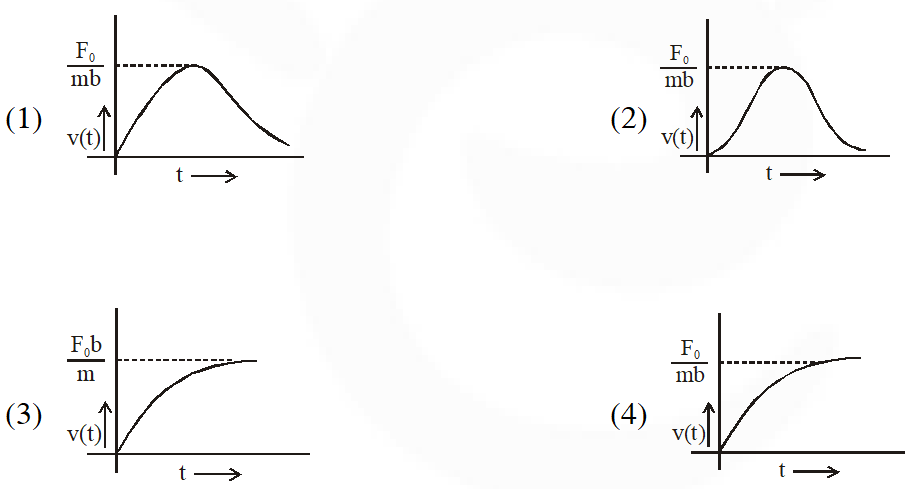[AIEEE - 2012]
Ans. (3) $\frac{\mathrm{d} \mathrm{v}}{\mathrm{dt}}=\frac{\mathrm{F}_{0}}{\mathrm{m}} \mathrm{e}^{-\mathrm{bt}}$ $\int_{0}^{\mathrm{v}} \mathrm{d} \mathrm{v}=\frac{\mathrm{F}_{0}}{\mathrm{m}} \int_{0}^{\mathrm{t}} \mathrm{e}^{-\mathrm{bt}} \mathrm{dt}$ $\mathrm{v}=\frac{-\mathrm{F}_{0}}{\mathrm{m} \mathrm{b}}\left[\mathrm{e}^{-\mathrm{bt}}-1\right]$ $=\frac{\mathrm{F}_{0}}{\mathrm{m} \mathrm{b}}\left[1-\mathrm{e}^{-\mathrm{bt}}\right]$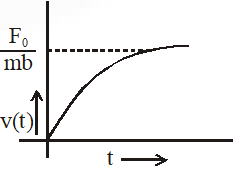Q. A projectile is given an initial velocity of $(\hat{i}+2 \hat{j}) m / s$ where $\hat{\mathbf{i}}$ is along the ground and $\hat{j}$ is along the vertical. If g = 10 m/s2, the equation of its trajectory is : (1) $\mathrm{y}=\mathrm{x}-5 \mathrm{x}^{2}$ (2) $\mathrm{y}=2 \mathrm{x}-5 \mathrm{x}^{2}$ (3) $4 y=2 x-5 x^{2}$ (4) $4 y=2 x-25 x^{2}$ [AIEEE - 2013]
Ans. (2) $\mathrm{u}=\hat{\mathrm{i}}+2 \hat{\mathrm{j}}$ $\mathrm{u}_{\mathrm{x}}=1$ $\mathrm{u}_{\mathrm{y}}=2$ $\tan \theta=\frac{2}{1}$ $\mathrm{y}=\mathrm{x} \tan \theta-\frac{1}{2} \mathrm{g} \frac{\mathrm{x}^{2}}{\mathrm{u}^{2} \cos ^{2} \theta}$ $\mathrm{y}=2 \mathrm{x}-5 \mathrm{x}^{2}$
Q. Two stones are thrown up simultaneously from the edge of a cliff 240 m high with initial speed of 10 m/s and 40 m/s respectively. Which of the following graph best represents the time variation of relative position of the second stone with respect to the first ? (Assume stones do not rebound after hitting the ground and neglect air resistance, take g = 10 $\mathrm{M} / \mathrm{S}^{2}$) (The figure are schematic and not drawn to scale)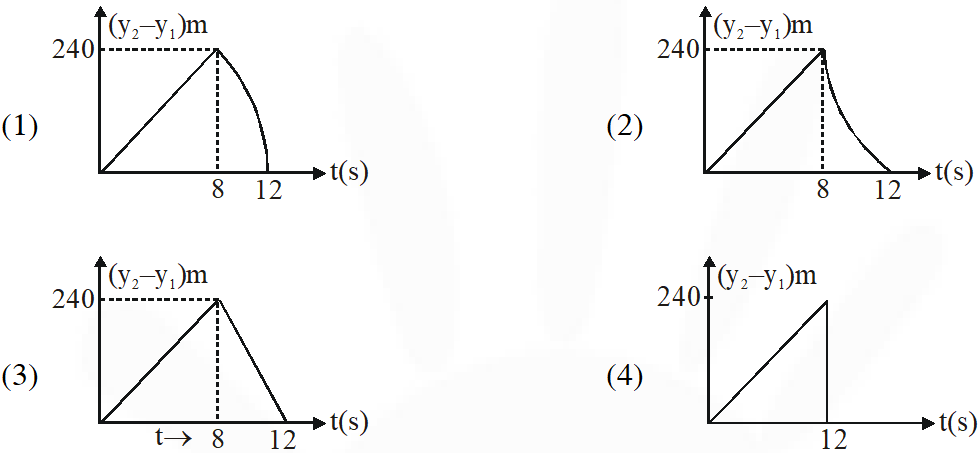[JEE Mains - 2015]
Ans. (1) $\mathrm{y}=2 \mathrm{x}-5 \mathrm{x}^{2}$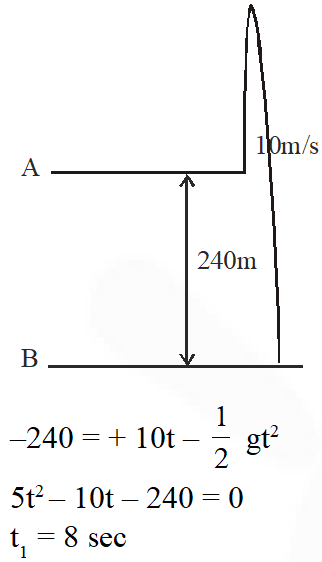For particle 2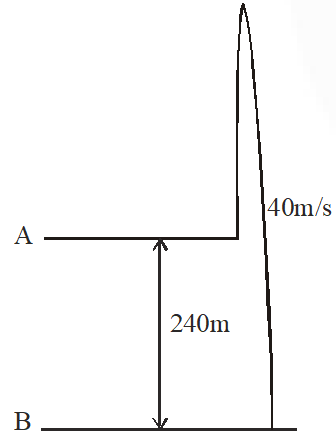$-240=40 \mathrm{t}-\frac{1}{2} \mathrm{gt}^{2}$ $5 t^{2}-40 t-240=0$ $\mathrm{t}_{2}=12 \mathrm{sec}$ for $0<\mathrm{t}<8$ sec $\rightarrow \mathrm{a}_{\text {rel }}=0$ straight line x-t graph for $8<\mathrm{t}<12$ sec $\rightarrow \mathrm{a}_{\mathrm{rel}}=-\mathrm{g}$ downward parabola for $\mathrm{t}>12 \mathrm{sec} \rightarrow$ Both particles comes to rest